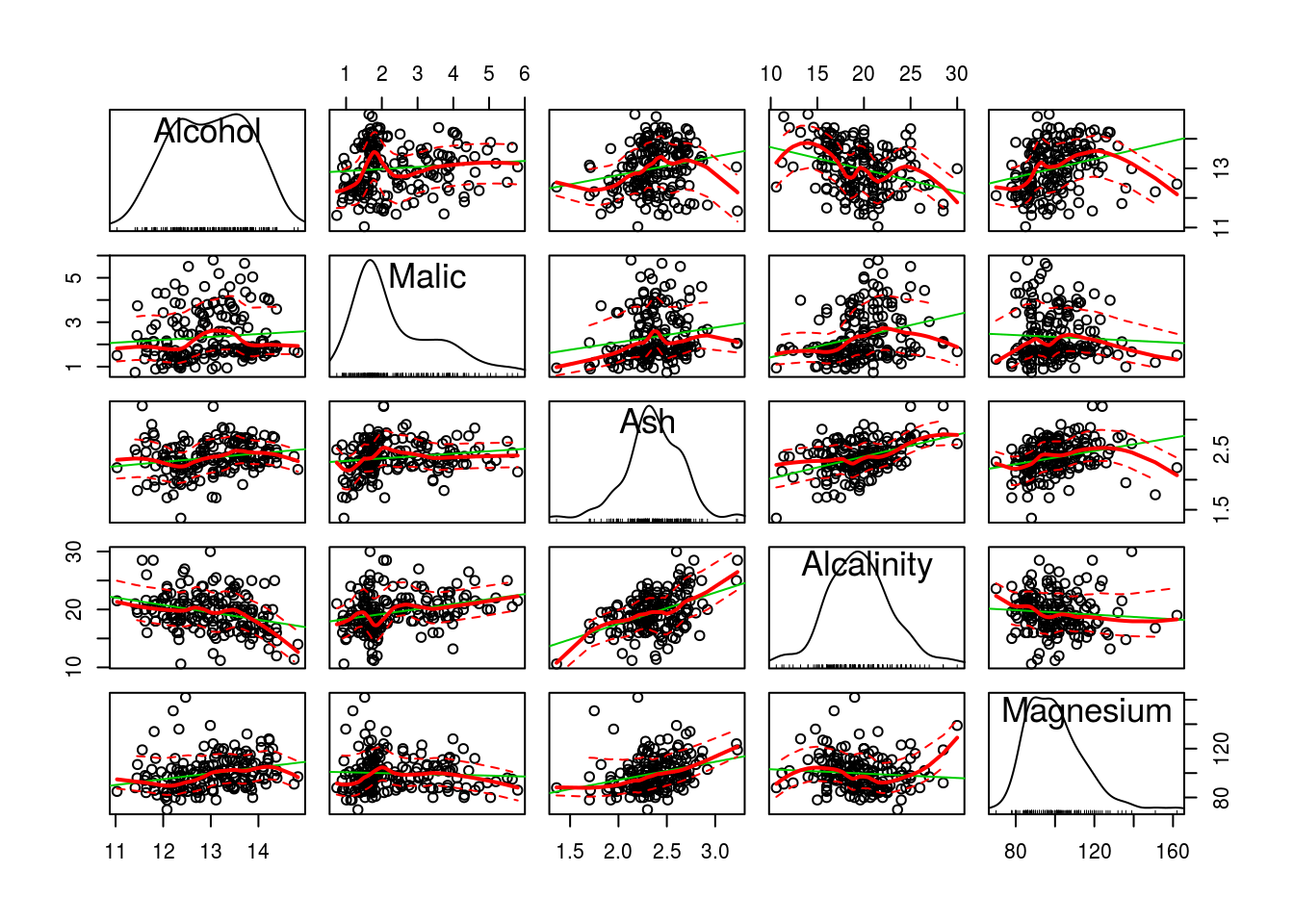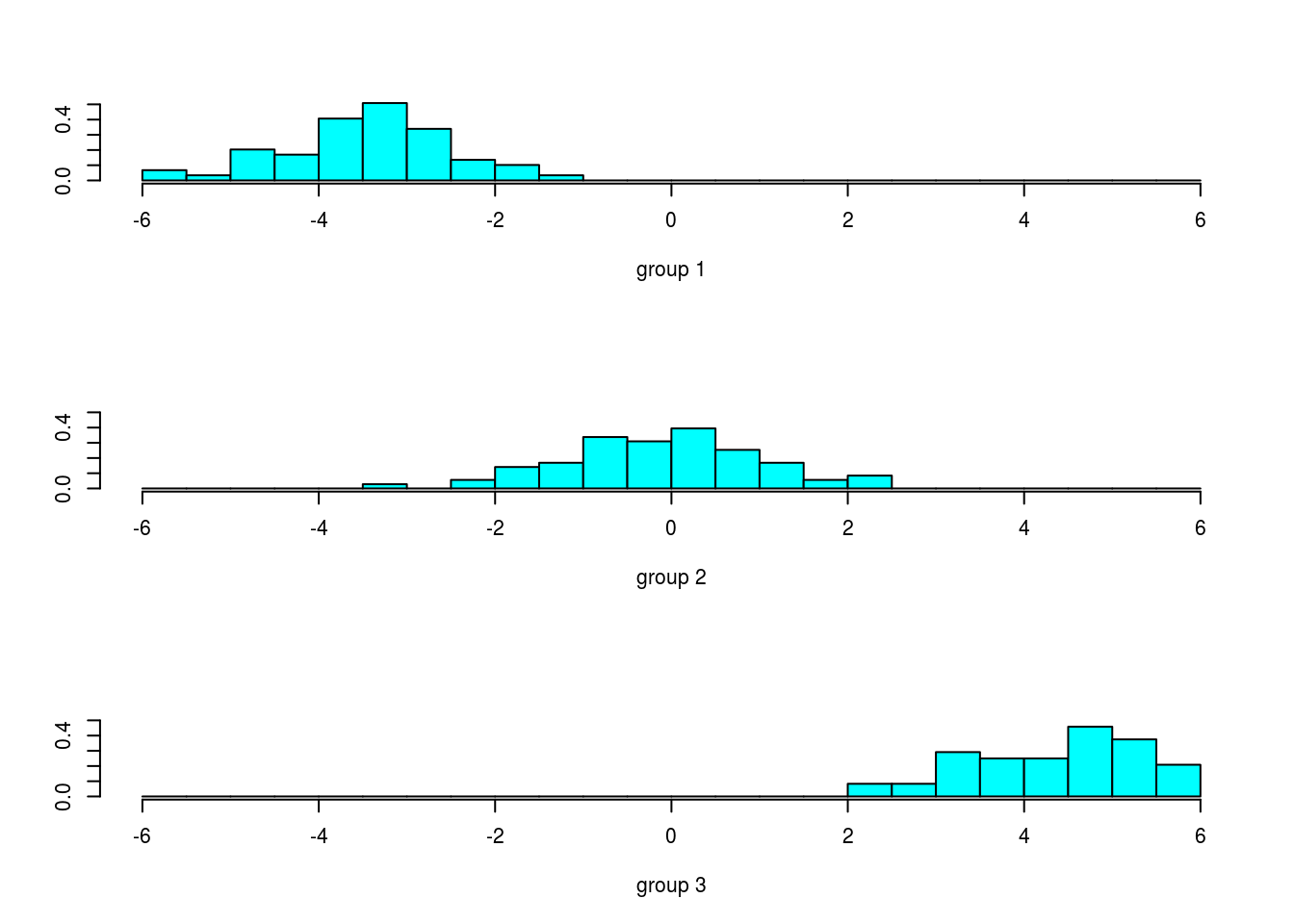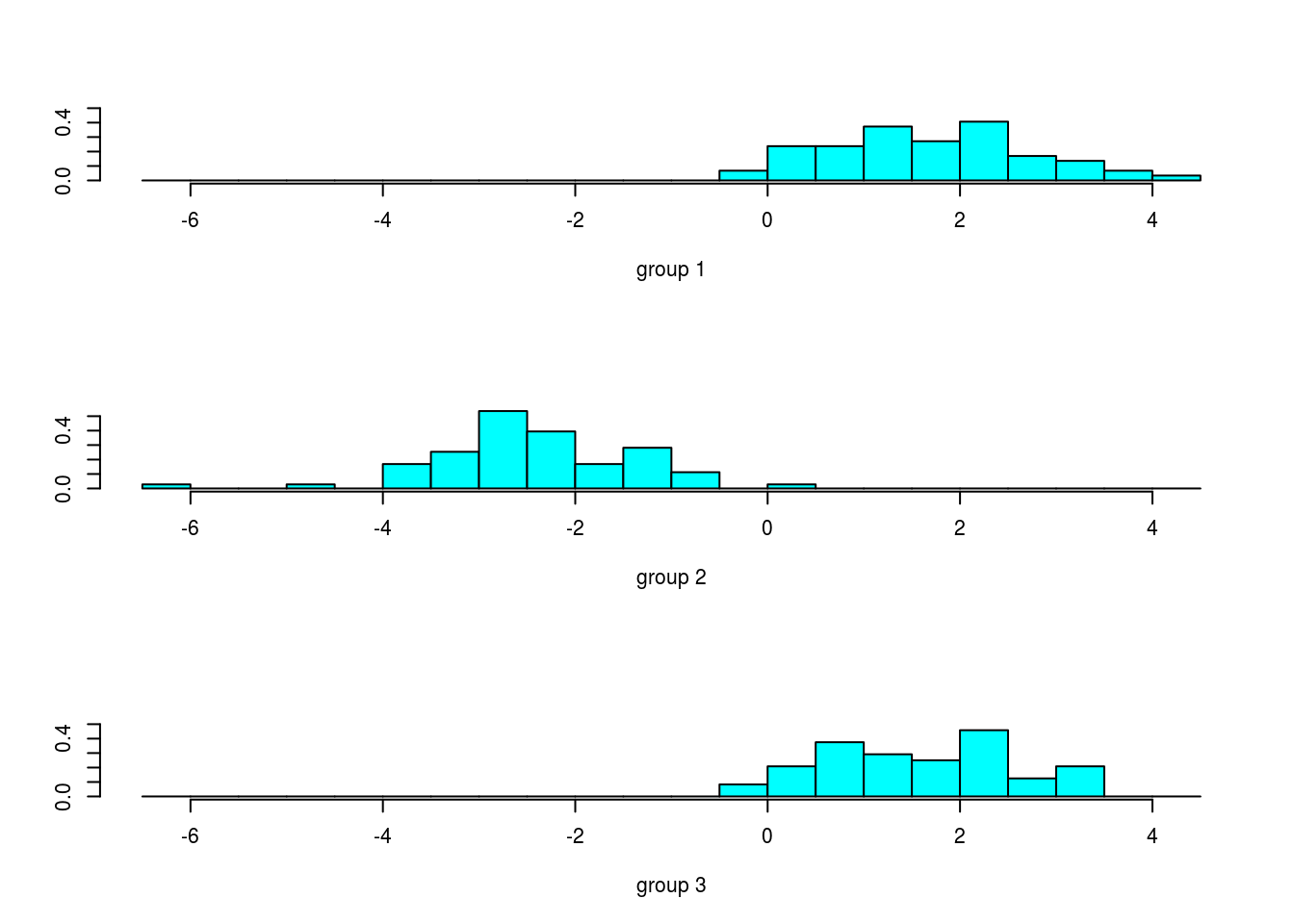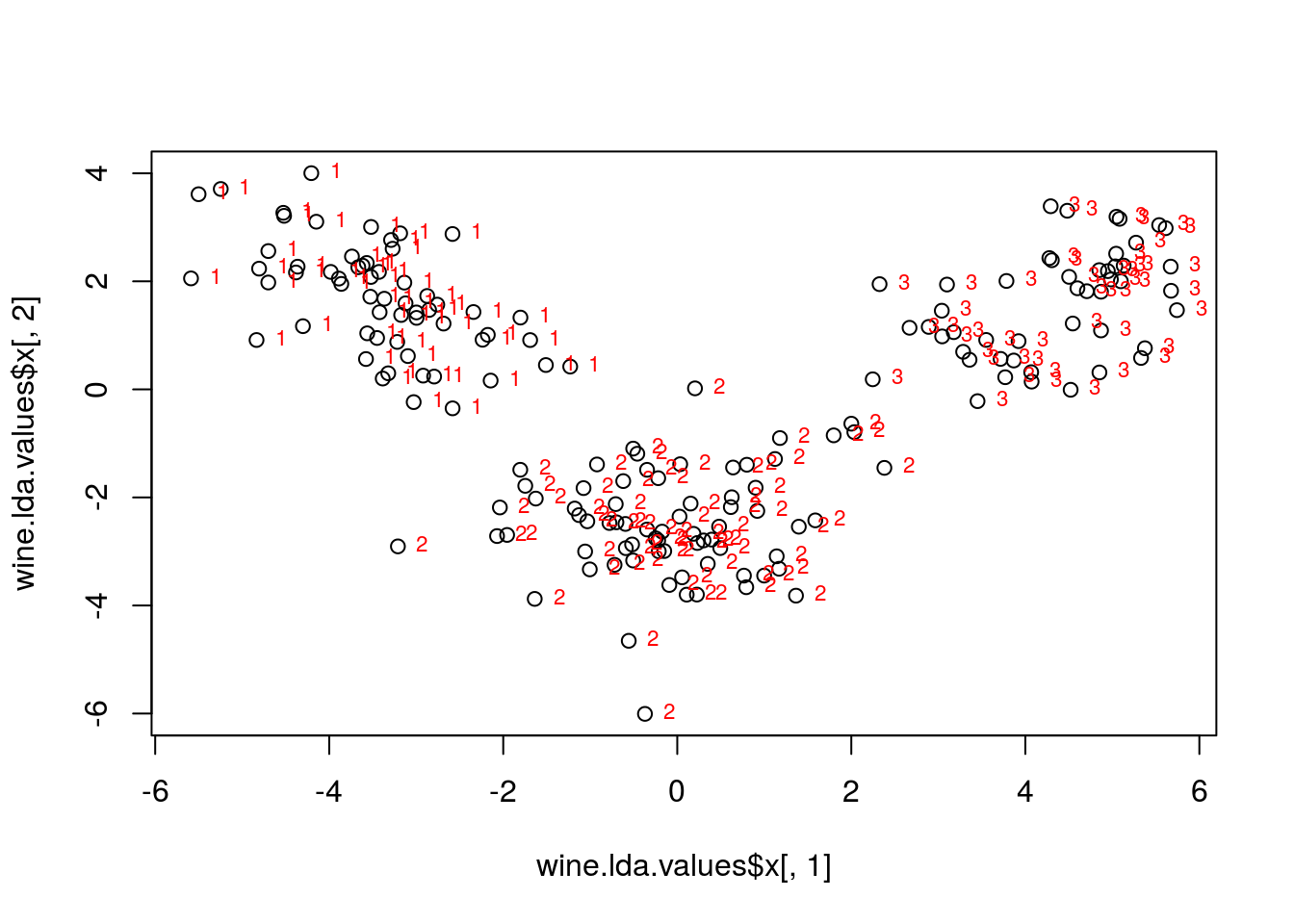### Brief notes on the theory of Discriminant Analysis

Linear discriminant analysis (LDA) and the related Fisher’s linear discriminant are methods used in statistics, pattern recognition and machine learning to find a linear combination of features which characterizes or separates two or more classes of objects or events. The resulting combination may be used as a linear classifier, or, more commonly, for dimensionality reduction before later classification.

Consider a set of observations $$x$$ (also called features, attributes, variables or measurements) for each sample of an object or event with known class $$y\in\{0,1\}$$. This set of samples is called the training set. The classification problem is then to find a good predictor for the class y of any sample of the same distribution (not necessarily from the training set) given only an observation $$x$$.

LDA approaches the problem by assuming that the conditional probability density functions $$p(x|y=1)$$ and $$p(x|y=2)$$ are both normally distributed with mean and covariance parameters $$(\mu_1, \Sigma_1)$$ and $$(\mu_2, \Sigma_2)$$, respectively. Under this assumption, the Bayes optimal solution is to predict points as being from the second class if the log of the likelihood ratios is below some threshold T, so that:

$( x- \mu_1)^T \Sigma_1^{-1} ( x- \mu_1) + \ln|\Sigma_1| - ( x- \mu_2)^T \Sigma_2^{-1} ( x- \mu_2) - \ln|\Sigma_2| < T$ Without any further assumptions, the resulting classifier is referred to as QDA (quadratic discriminant analysis). When $$\Sigma_1=\Sigma_2=\Sigma$$ (homoscedasticity assumption) the discriminant functions it is:

$( x- \mu_1)^T \Sigma^{-1} ( x- \mu_1) - ( x- \mu_2)^T \Sigma^{-1} ( x- \mu_2) < T,$

this is the expression for the LDA (linear discriminant analysis) function. In this case, several terms cancel and the above decision criterion it is linear: $w \cdot x > c,$ for some threshold constant c.

#### Fisher’s linear discriminant

The terms Fisher’s linear discriminant and LDA are often used interchangeably, although Fisher’s original article actually describes a slightly different discriminant, which does not make some of the assumptions of LDA such as normally distributed classes or equal class covariances.

Suppose two classes of observations have means $$\mu_1, \mu_2$$ and covariances $$\Sigma_1$$ and $$\Sigma_2$$. Then the linear combination of features $$w \cdot x$$ will have means $$w \cdot \mu_i$$ and variances $$w ^T \Sigma_i w$$ for $$i=1,2$$. Fisher defined the separation between these two distributions to be the ratio of the variance between the classes to the variance within the classes:

$S=\frac{\sigma_{\text{between}}^2}{\sigma_{\text{within}}^2}= \frac{( w \cdot \mu_2 - w \cdot \mu_1)^2}{ w^T \Sigma_2 w + w^T \Sigma_1 w} = \frac{( w \cdot ( \mu_2 - \mu_1))^2}{ w^T (\Sigma_1+\Sigma_2) w}$ This measure is, in some sense, a measure of the signal-to-noise ratio for the class labeling. It can be shown that the maximum separation occurs when

$w \propto (\Sigma_1+\Sigma_2)^{-1}( \mu_2 - \mu_1)$

When the assumptions of LDA are satisfied, the above equation is equivalent to LDA.

Be sure to note that the vector $$w$$ is the normal to the discriminant hyperplane. As an example, in a two dimensional problem, the line that best divides the two groups is perpendicular to $$w$$.

Generally, the data points to be discriminated are projected onto $$w$$; then the threshold that best separates the data is chosen from analysis of the one-dimensional distribution. There is no general rule for the threshold. However, if projections of points from both classes exhibit approximately the same distributions, a good choice would be the hyperplane between projections of the two means, $$w \cdot \mu_1$$ and $$w \cdot \mu_2$$. In this case the parameter c in threshold condition $$w\cdot x>c$$ can be found explicitly:

$c = w \cdot \frac{1}{2} ( \mu_1 + \mu_2) = \frac{1}{2} \mu_2^t \Sigma^{-1} \mu_2 - \frac{1}{2} \mu_1^t \Sigma^{-1} \mu_1$

##### Possible applications:
• Bankruptcy prediction: In bankruptcy prediction based on accounting ratios and other financial variables, linear discriminant analysis was the first statistical method applied to systematically explain which firms entered bankruptcy vs. survived.

• Marketing: In marketing, discriminant analysis was once often used to determine the factors which distinguish different types of customers and/or products on the basis of surveys or other forms of collected data.
• Biomedical studies: The main application of discriminant analysis in medicine is the assessment of severity state of a patient and prognosis of disease outcome. For example, during retrospective analysis, patients are divided into groups according to severity of disease - mild, moderate and severe form. Then results of clinical and laboratory analyses are studied in order to reveal variables which are statistically different in studied groups. Using these variables, discriminant functions are built which help to objectively classify disease in a future patient into mild, moderate or severe form.

### Study Case I: Wine classification

In this first study case, the wine data set, we have 13 chemical concentrations describing wine samples from three cultivars.

library(car)
# install.packages('rattle')
data(wine, package='rattle')
attach(wine)
head(wine)
##   Type Alcohol Malic  Ash Alcalinity Magnesium Phenols Flavanoids
## 1    1   14.23  1.71 2.43       15.6       127    2.80       3.06
## 2    1   13.20  1.78 2.14       11.2       100    2.65       2.76
## 3    1   13.16  2.36 2.67       18.6       101    2.80       3.24
## 4    1   14.37  1.95 2.50       16.8       113    3.85       3.49
## 5    1   13.24  2.59 2.87       21.0       118    2.80       2.69
## 6    1   14.20  1.76 2.45       15.2       112    3.27       3.39
##   Nonflavanoids Proanthocyanins Color  Hue Dilution Proline
## 1          0.28            2.29  5.64 1.04     3.92    1065
## 2          0.26            1.28  4.38 1.05     3.40    1050
## 3          0.30            2.81  5.68 1.03     3.17    1185
## 4          0.24            2.18  7.80 0.86     3.45    1480
## 5          0.39            1.82  4.32 1.04     2.93     735
## 6          0.34            1.97  6.75 1.05     2.85    1450
scatterplotMatrix(wine[2:6])The purpose of linear discriminant analysis (LDA) in this example is to find the linear combinations of the original variables (the 13 chemical concentrations here) that gives the best possible separation between the groups (wine cultivars here) in our data set. Linear discriminant analysis is also known as “canonical discriminant analysis”, or simply “discriminant analysis”.

If we want to separate the wines by cultivar, the wines come from three different cultivars, so the number of groups $$G = 3$$, and the number of variables is 13 (13 chemicals’ concentrations; $$p = 13$$). The maximum number of useful discriminant functions that can separate the wines by cultivar is the minimum of $$G-1$$ and $$p$$, and so in this case it is the minimum of 2 and 13, which is 2. Thus, we can find at most 2 useful discriminant functions to separate the wines by cultivar, using the 13 chemical concentration variables.

You can carry out a linear discriminant analysis using the “lda()” function from the R MASS package. To use this function, we first need to install the MASS R package.

# install.packages('MASS')
library(MASS)
wine.lda <- lda(Type ~ ., data=wine)

To get the values of the loadings of the discriminant functions for the wine data, we can type:

wine.lda
## Call:
## lda(Type ~ ., data = wine)
##
## Prior probabilities of groups:
##         1         2         3
## 0.3314607 0.3988764 0.2696629
##
## Group means:
##    Alcohol    Malic      Ash Alcalinity Magnesium  Phenols Flavanoids
## 1 13.74475 2.010678 2.455593   17.03729  106.3390 2.840169  2.9823729
## 2 12.27873 1.932676 2.244789   20.23803   94.5493 2.258873  2.0808451
## 3 13.15375 3.333750 2.437083   21.41667   99.3125 1.678750  0.7814583
##   Nonflavanoids Proanthocyanins    Color       Hue Dilution   Proline
## 1      0.290000        1.899322 5.528305 1.0620339 3.157797 1115.7119
## 2      0.363662        1.630282 3.086620 1.0562817 2.785352  519.5070
## 3      0.447500        1.153542 7.396250 0.6827083 1.683542  629.8958
##
## Coefficients of linear discriminants:
##                          LD1           LD2
## Alcohol         -0.403399781  0.8717930699
## Malic            0.165254596  0.3053797325
## Ash             -0.369075256  2.3458497486
## Alcalinity       0.154797889 -0.1463807654
## Magnesium       -0.002163496 -0.0004627565
## Phenols          0.618052068 -0.0322128171
## Flavanoids      -1.661191235 -0.4919980543
## Nonflavanoids   -1.495818440 -1.6309537953
## Proanthocyanins  0.134092628 -0.3070875776
## Color            0.355055710  0.2532306865
## Hue             -0.818036073 -1.5156344987
## Dilution        -1.157559376  0.0511839665
## Proline         -0.002691206  0.0028529846
##
## Proportion of trace:
##    LD1    LD2
## 0.6875 0.3125

This means that the first discriminant function is a linear combination of the variables: $$-0.403*Alcohol + 0.165*Malic \dots - 0.003*Proline$$. For convenience, the value for each discriminant function (eg. the first discriminant function) are scaled so that their mean value is zero and its variance is one.

The “proportion of trace” that is printed when you type “wine.lda” (the variable returned by the lda() function) is the percentage separation achieved by each discriminant function. For example, for the wine data we get the same values as just calculated (68.75% and 31.25%).

###### A Stacked Histogram of the LDA Values

A nice way of displaying the results of a linear discriminant analysis (LDA) is to make a stacked histogram of the values of the discriminant function for the samples from different groups (different wine cultivars in our example).

We can do this using the “ldahist()” function in R. For example, to make a stacked histogram of the first discriminant function’s values for wine samples of the three different wine cultivars, we type:

wine.lda.values <- predict(wine.lda)
ldahist(data = wine.lda.values$x[,1], g=Type)We therefore investigate whether the second discriminant function separates those cultivars, by making a stacked histogram of the second discriminant function’s values: ldahist(data = wine.lda.values$x[,2], g=Type)##### Scatterplots of the Discriminant Functions

We can obtain a scatterplot of the best two discriminant functions, with the data points labelled by cultivar, by typing:

plot(wine.lda.values$x[,1],wine.lda.values$x[,2]) # make a scatterplot
text(wine.lda.values$x[,1],wine.lda.values$x[,2],Type,cex=0.7,pos=4,col="red") # add labelsFrom the scatterplot of the first two discriminant functions, we can see that the wines from the three cultivars are well separated in the scatterplot. The first discriminant function (x-axis) separates cultivars 1 and 3 very well, but doesn’t not perfectly separate cultivars 1 and 3, or cultivars 2 and 3.

The second discriminant function (y-axis) achieves a fairly good separation of cultivars 1 and 3, and cultivars 2 and 3, although it is not totally perfect.

To achieve a very good separation of the three cultivars, it would be best to use both the first and second discriminant functions together, since the first discriminant function can separate cultivars 1 and 3 very well, and the second discriminant function can separate cultivars 1 and 2, and cultivars 2 and 3, reasonably well. ### Study Case II: MBA admission data

The dataset provide admission data for applicants to graduate schools in business. The objective is to use the GPA and GMAT scores to predict the likelihood of admission (admit, notadmit, and borderline).

url <- 'http://www.biz.uiowa.edu/faculty/jledolter/DataMining/admission.csv'

admit <- read.csv(url)

head(admit)
##    GPA GMAT    De
## 1 2.96  596 admit
## 2 3.14  473 admit
## 3 3.22  482 admit
## 4 3.29  527 admit
## 5 3.69  505 admit
## 6 3.46  693 admit

We can make a scatterplot with the data at hand:

adm=data.frame(admit)

plot(adm$GPA,adm$GMAT,col=adm\$De)